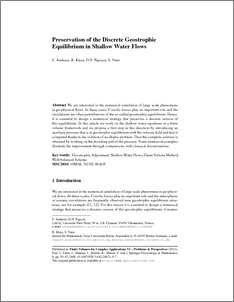Repository: Freie Universität Berlin, Math Department

# Preservation of the discrete geostrophic equilibrium in shallow water flows

Audusse, E. and Klein, R. and Nguyen, D.D. and Vater, S. (2011) Preservation of the discrete geostrophic equilibrium in shallow water flows. In: Finite Volumes for Complex Applications VI - Problems& Perspectives, 6.6.-10.6. 2011, Prague, Czech Republic.Preview

4MB

Official URL: http://dx.doi.org/10.1007/978-3-642-20671-9_7

## Abstract

We are interested in the numerical simulation of large scale phenomena in geophysical flows. In these cases, Coriolis forces play an important role and the circulations are often perturbations of the so-called geostrophic equilibrium. Hence, it is essential to design a numerical strategy that preserves a discrete version of this equilibrium. In this article we work on the shallow water equations in a finite volume framework and we propose a first step in this direction by introducing an auxiliary pressure that is in geostrophic equilibrium with the velocity field and that is computed thanks to the solution of an elliptic problem. Then the complete solution is obtained by working on the deviating part of the pressure. Some numerical examples illustrate the improvement through comparisons with classical discretizations.the deviating part of the pressure. Some numerical examples illustrate the improvement when compare with classical finite volume methods.

Item Type: Conference or Workshop Item (Paper) Mathematical and Computer Sciences > Mathematics > Applied Mathematics Department of Mathematics and Computer Science > Institute of Mathematics > Geophysical Fluid Dynamics Group 1034 Ulrike Eickers 09 Feb 2011 16:03 03 Mar 2017 14:41

Repository Staff Only: item control page